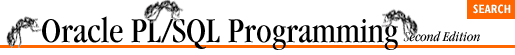#Chapter 7Loops## 7.5 The WHILE Loop

The WHILE loop is a conditional loop that continues to execute as long as the Boolean condition defined in the loop boundary evaluates to TRUE. Because the WHILE loop execution depends on a condition and is not fixed, use a WHILE loop if you don't know ahead of time the number of times a loop must execute.

Here is the general syntax for the WHILE loop:

```WHILE <condition>
LOOP
<executable statement(s)>
END LOOP;```

where <condition> is a Boolean variable or an expression that evaluates to a Boolean value of TRUE, FALSE, or NULL. Each time an iteration of the loop's body is to be executed, the condition is checked. If it evaluates to TRUE, then the body is executed. If it evaluates to FALSE or to NULL, then the loop terminates and control passes to the next executable statement following the END LOOP statement.

The following table summarizes the properties of the WHILE loop:

Property

Description

How the loop is terminated

The WHILE loop terminates when the Boolean expression in its boundary evaluates to FALSE or NULL.

When the test for termination takes place

The test for termination of a WHILE loop takes place in the loop boundary. This evaluation occurs prior to the first and each subsequent execution of the body. The WHILE loop, therefore, cannot be guaranteed to always execute its loop even a single time.

Reason to use this loop

Use the WHILE loop when:

• You are not sure how many times you must execute the loop body, and

• You will want to conditionally terminate the loop, and

• You don't have to execute the body at least one time.

The WHILE loop's condition is tested at the beginning of the loop's iteration, before the body of the loop is executed. There are two consequences to this pre-execution test:

• All the information needed to evaluate the condition must be set before the loop is executed for the first time.

• It is possible that the WHILE loop will not execute even a single time.

### 7.5.1 The Infinite WHILE Loop

One of the dangers of the simple loop is that it could be an infinite loop if the body of the loop never executes an EXIT statement. While this is less of a problem with the WHILE loop, you should be aware that it is certainly possible to construct a WHILE loop that is syntactically equivalent to the infinite LOOP. The most obvious version of an infinite WHILE loop is the following:

```WHILE TRUE
LOOP
<executable statement(s)>
END LOOP;```

Sometimes, however, an infinite WHILE loop can be disguised by poor programming techniques. The following WHILE loop will terminate only when end_of_analysis is set to TRUE:

```WHILE NOT end_of_analysis
LOOP
perform_analysis;
get_next_record;
IF analysis_cursor%NOTFOUND AND
next_analysis_step IS NULL
THEN
end_of_analysis := TRUE;
END IF;
END LOOP;```

In this WHILE loop, the end_of_analysis Boolean variable is set to TRUE only if the analysis_cursor fetches no data and we are at the last step of the analysis.

Unfortunately, both the cursor and the next_analysis_step variable are completely invisible in the loop itself. How is next_analysis_step set? Where is the cursor declared? How is a record fetched? This is a very dangerous way to structure code because if you do fall into an infinite loop, the information you need to resolve the problem is not readily available.